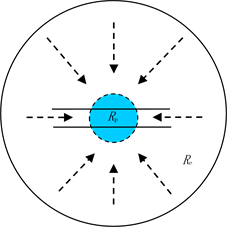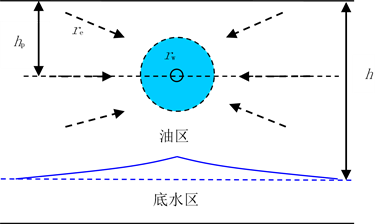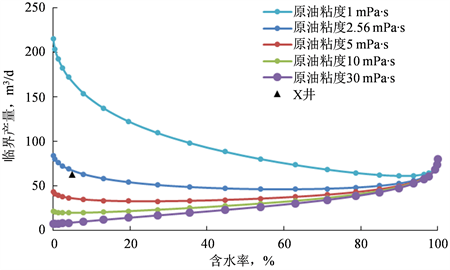# 注水开发底水油藏水平井预警产量计算新方法A Calculation Method of Warning Production of Horizontal Well in Water-Flooding Bottom Water Reservoir

• 全文下载: PDF(563KB)    PP.1-6   DOI: 10.12677/APF.2018.81001
• 下载量: 560  浏览量: 1,746

The warning production of the horizontal well in the bottom water reservoir is an important pa-rameter to postpone or avoid the bottom water coning. The present method is only suitable for single phase flow. And there is no calculation method for warning output of horizontal well in bottom water reservoir with water injection. Therefore, the 3D percolation field of horizontal well is approximately divided into two parts including internal and external percolation fields. According to the theory of plane radial flow, the warning output of each seepage field is calculated respectively. Then the warning output of the horizontal well is obtained by the superposition of the theory of equal value seepage. In the end, a new method for calculating the warning output of horizontal wells at different water content stages is established by combining with the phase seepage curve. The calculation results show that when the crude oil viscosity is relatively small, the warning yield decreases first and then increases with the increase of water content. When the viscosity of crude oil is larger, the warning output increases with the increase of water content, so the liquid can be properly carried out after the injection of water. It is applied in an offshore oilfield. The calculated results are basically the same with the numerical simulation results. The feasibility of the method is verified. It is recommended that the daily production of X well be cut down when the water content is over 15%.

1. 引言

2. 水平井渗流场解析

3. 水平井预警产量计算

3.1. 注入水突破前预警产量计算

${q}_{\text{c1}}=J\cdot \Delta p=\frac{2\text{π}{k}_{\text{h}}h}{{B}_{\text{o}}{\mu }_{\text{o}}\left(\mathrm{ln}\frac{{R}_{\text{e}}}{{R}_{\text{p}}}+s\right)}\cdot \frac{\Delta {\rho }_{\text{ow}}g\left({h}^{2}-{h}_{\text{p}}^{2}\right)}{2h}$ (1)Figure 1. Transversal section seepage field of horizontal wellFigure 2. Longitudinal section seepage field of horizontal well

${q}_{\text{c2}}=\frac{2\text{π}{k}_{\text{v}}L}{{B}_{\text{o}}{\mu }_{\text{o}}\left(\mathrm{ln}\frac{{r}_{\text{e}}}{{r}_{\text{w}}}+s\right)}\cdot \Delta p$ (2)

$\Delta p=\frac{\Delta {\rho }_{\text{ow}}g\left({h}^{2}-{h}_{\text{p}}^{2}\right)}{2h}$ (3)

${q}_{\text{c2}}=\frac{\pi {k}_{\text{v}}L\Delta {\rho }_{\text{ow}}g\left({h}^{2}-{h}_{\text{p}}^{2}\right)}{{B}_{\text{o}}{\mu }_{\text{o}}h\left(\mathrm{ln}\frac{{r}_{\text{e}}}{{r}_{\text{w}}}+s\right)}$ (4)

${q}_{\text{c}}=\frac{\text{π}{k}_{\text{h}}\Delta {\rho }_{\text{ow}}g\left({h}^{2}-{h}_{\text{p}}^{2}\right)}{{B}_{\text{o}}{\mu }_{\text{o}}\left(\mathrm{ln}\frac{{R}_{\text{e}}}{{R}_{\text{p}}}+s\right)}+\frac{\text{π}{k}_{\text{v}}L\Delta {\rho }_{\text{ow}}g\left({h}^{2}-{h}_{\text{p}}^{2}\right)}{{B}_{\text{o}}{\mu }_{\text{o}}h\left(\mathrm{ln}\frac{{r}_{\text{e}}}{{r}_{\text{w}}}+s\right)}$ (5)

3.2. 注入水突破后预警产量计算

${q}_{\text{c1}}=\frac{\text{π}{k}_{\text{h}}\Delta {\rho }_{\text{ow}}g\left({h}^{2}-{h}_{\text{p}}^{2}\right)}{\mathrm{ln}\frac{{R}_{\text{e}}}{{R}_{\text{p}}}+s}\left(\frac{{k}_{\text{ro}}}{{B}_{\text{o}}{\mu }_{\text{o}}}+\frac{{k}_{\text{rw}}}{{B}_{\text{w}}{\mu }_{\text{w}}}\right)$ (6)

${q}_{\text{c2}}=\frac{\text{π}{k}_{\text{v}}L\Delta {\rho }_{\text{ow}}g\left({h}^{2}-{h}_{\text{p}}^{2}\right)}{h\left(\mathrm{ln}\frac{{r}_{\text{e}}}{{r}_{\text{w}}}+s\right)}\left(\frac{{k}_{\text{ro}}}{{B}_{\text{o}}{\mu }_{\text{o}}}+\frac{{k}_{\text{rw}}}{{B}_{\text{w}}{\mu }_{\text{w}}}\right)$ (7)

${q}_{\text{c}}=\text{π}{k}_{\text{h}}\Delta {\rho }_{\text{ow}}g\left({h}^{2}-{h}_{\text{p}}^{2}\right)\left[\frac{{k}_{\text{h}}}{\mathrm{ln}\frac{{R}_{\text{e}}}{{R}_{\text{p}}}+s}+\frac{{k}_{\text{v}}L}{h\left(\mathrm{ln}\frac{{r}_{\text{e}}}{{r}_{\text{w}}}+s\right)}\right]\left(\frac{{k}_{\text{ro}}}{{B}_{\text{o}}{\mu }_{\text{o}}}+\frac{{k}_{\text{rw}}}{{B}_{\text{w}}{\mu }_{\text{w}}}\right)$ (8)

① 将相渗曲线上含水饱和度对应的油水相渗值代入分流量方程  ，间接建立油水相渗值与含水率的关系：

${f}_{\text{w}}=\frac{1}{1+\frac{{k}_{\text{ro}}}{{k}_{\text{rw}}}\frac{{\mu }_{\text{w}}}{{\mu }_{\text{o}}}}$ (9)

② 将采油井实际的含水率代入①所建立的关系反求对应的油水相渗值；

③ 将油水相渗值代入式(8)计算对应含水率下的预警产量。

4. 实例计算分析

5. 结论

1) 将水平井的三维渗流场进行近似分解，建立了注水开发底水油藏水平井预警产量的计算方法，该Table 1. Basic parameter table of horizontal wellFigure 3. The phase seepage curve of an oil fieldFigure 4. Early warning output chart of different water content stages of different crude oil viscosity

2) 不同原油粘度下底水油藏的预警产量随着含水率的变化规律不同。原油粘度较小时，预警产量随着含水率的增大先减小后增大，为避免底水脊进注入水突破后要对应调整工作制度；原油粘度较大时，预警产量随着含水率的增大不断增大，故注入水突破后可适当开展提液。

3) 矿场应用结果与数值模拟结果一致，验证了方法的可行性，对相似油田具有重要借鉴及指导意义。

NOTES

*通讯作者。

  王家禄, 刘玉章, 江如意, 等. 水平井开采底水油藏水脊脊进规律的物理模拟[J]. 石油勘探与开发, 2007, 34(5): 590-593.  吉兰敏, 姜汉桥, 陈民锋, 等. 非均质底水油藏临界产量预测[J]. 大庆石油地质与开发, 2009, 28(6): 119-124.  Chaperon, I. (1986) Theoretical Study of Coning toward Horizontal and Vertical well in Anisotropic Formations: Subcritical and Critical Rates. SPE15377.  Giger, F.M. (1989) Analytic Two-Dimension Models of Water Cresting before Breakthrough for Horizontal Wells. SPE Reservoir Evaluation & Engineering, 4, 409-416. https://doi.org/10.2118/15378-PA  Joshi, S.D. (1991) Horizontal Well Technology. Penn Well Publishing Company, Tulsa, OK, 59-105.  Guo, B.Y. and Lee, R.L. (1992) Determination of the Maximum Water-Free Production Rate of a Horizontal Well with Water-Oil-Interface Cresting. SPE24324.  范子菲, 林志芳. 底水驱动油藏水平井临界产量公式及其变化规律研究[J]. 石油勘探与开发, 1994, 21(1): 65-70.  窦宏恩. 水平井开采底水油藏临界产量的计算[J]. 石油钻采工艺, 1997, 19(3): 70-75.  周代余, 江同文, 赵金洲, 等. 底水油藏水平井临界产量及其不确定性分析[J]. 钻采工艺, 2005, 28(1): 33-36.  姚凯, 陈树营, 姜汉桥, 等. 水驱油藏水平井临界产量的研究与应用[J]. 油气地质与采收率, 2009, 16(2): 77-80.  陈元千. 预测水锥和气锥水平井临界产量的新方法[J]. 中国海上油气, 2010, 22(1): 22-26.  袁淋, 李晓平, 延懿宸. 底水油藏水平井临界产量确定新方法[J]. 天然气与石油, 2015, 33(1): 65-68.  涂彬, 韩洁, 孙键. 厚层底水油藏油井临界产量计算方法[J]. 石油钻探技术, 2014, 42(4): 107-110.  李传亮. 修正Dupuit临界产量公式[J]. 石油勘探与开发, 1993, 20(4): 91-95.  姜汉桥, 姚军, 姜瑞忠. 油藏工程原理与方法[M]. 东营: 中国石油大学出版社, 2006: 61.